Suppose that a virtual world has been defined with millions of triangles. If two complicated, nonconvex bodies are to be checked for collision, then the computational cost may be high. For this complicated situation, collision detection often becomes a two-phase process:

1. Broad Phase: In the broad phase, the task is to avoid performing expensive computations for bodies that are far away from each other. Simple bounding boxes can be placed around each of the bodies, and simple tests can be performed to avoid costly collision checking unless the boxes intersect. Hashing schemes can be employed in some cases to greatly reduce the number of pairs of boxes that have to be tested for intersect .
2. Narrow Phase: In the narrow phase individual pairs of model parts are each checked carefully for collision. This involves the expensive tests, such as triangle-triangle intersection.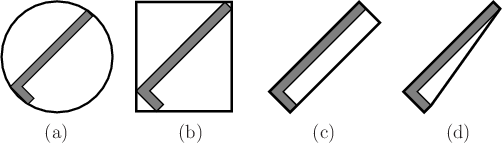In the broad phase, hierarchical methods generally decompose each body into a tree. Each vertex in the tree represents a bounding region that contains some subset of the body. The bounding region of the root vertex contains the whole body. Two opposing criteria that guide the selection of the type of bounding region:

1. The region should fit the intended model points as tightly as possible.
2. The intersection test for two regions should be as efficient as possible.
Several popular choices are shown in Figure 8.11, for the case of an L-shaped body. Hierarchical methods are also useful for quickly eliminating many triangles from consideration in visual rendering, as mentioned in Section 7.1.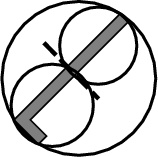The tree is constructed for a body,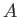(or alternatively,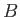) recursively as follows. For each vertex, consider the set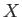of all points inthat are contained in the bounding region. Two child vertices are constructed by defining two smaller bounding regions whose union covers. The split is made so that the portion covered by each child is of similar size. If the geometric model consists of primitives such as triangles, then a split could be made to separate the triangles into two sets of roughly the same number of triangles. A bounding region is then computed for each of the children. Figure 8.12 shows an example of a split for the case of an L-shaped body. Children are generated recursively by making splits until very simple sets are obtained. For example, in the case of triangles in space, a split is made unless the vertex represents a single triangle. In this case, it is easy to test for the intersection of two triangles.

Consider the problem of determining whether bodiesandare in collision. Suppose that the treesand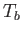have been constructed forand, respectively. If the bounding regions of the root vertices ofanddo not intersect, then it is known thatandare not in collision without performing any additional computation. If the bounding regions do intersect, then the bounding regions of the children ofare compared to the bounding region of. If either of these intersect, then the bounding region ofis replaced with the bounding regions of its children, and the process continues recursively. As long as the bounding regions intersect, lower levels of the trees are traversed, until eventually the leaves are reached. At the leaves the algorithm tests the individual triangles for collision, instead of bounding regions. Note that as the trees are traversed, if a bounding region from the vertex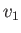ofdoes not intersect the bounding region from a vertex,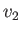, of, then no children ofhave to be compared to children of. Usually, this dramatically reduces the number of comparisons, relative to a naive approach that tests all pairs of triangles for intersection.

Steven M LaValle 2020-11-11